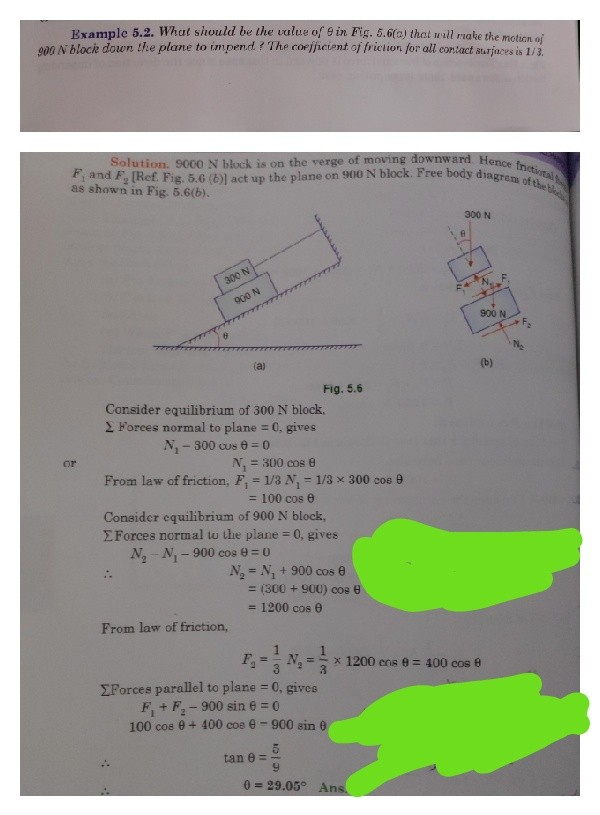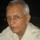Coffee Room
Discuss anything here - everything that you wish to discuss with fellow engineers.
12890 Members
Join this group to post and comment.# Since tan of the angle of respose is equal to the coefficient of friction. Why can't we find the angle of repose by simply finding the tan inverse of coefficient of friction.?Since tan of the angle of respose is equal to the coefficient of friction. Why can't we find the angle of repose by simply finding the tan inverse of coefficient of friction.Because this is the coefficient of internal friction of particulate materials. This depends a lot on surface contamination, humidity and such. Just consider getting the value of the coefficient for materials like mustard seeds, wheat, rice and many such materials.

I did a lot of work on the measurement of repose angle of both organic and inorganic particles at IIT Madras in the early 1960s.

The gadget designed was a simple transparent top open narrow rectangular cell of acrylic. The material was slowly poured into the gap, where it formed a neat triangular heap. It was a no-brainer to measure the base angle with a regular protractor. Took just a very few minutes for getting the value for any materials even with a large number of measurements to get a good mean value.

Share this content on your social channels -